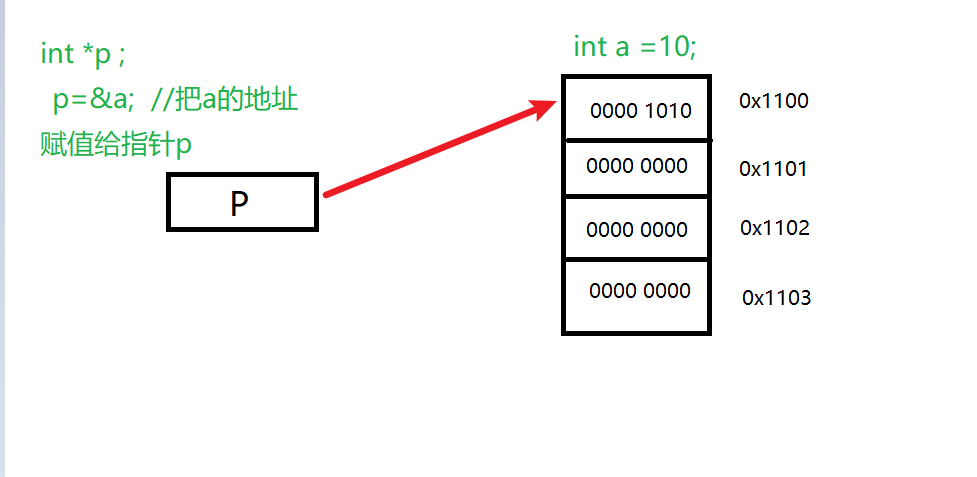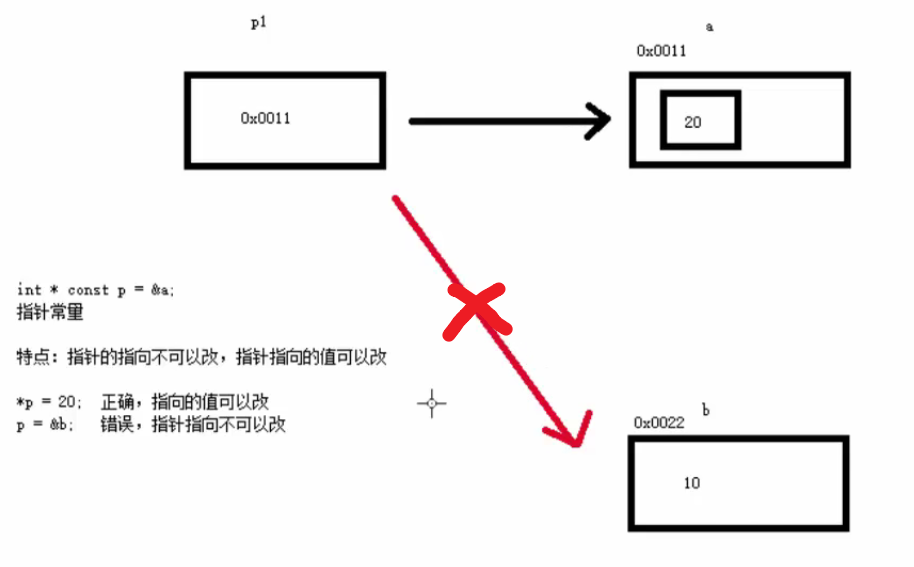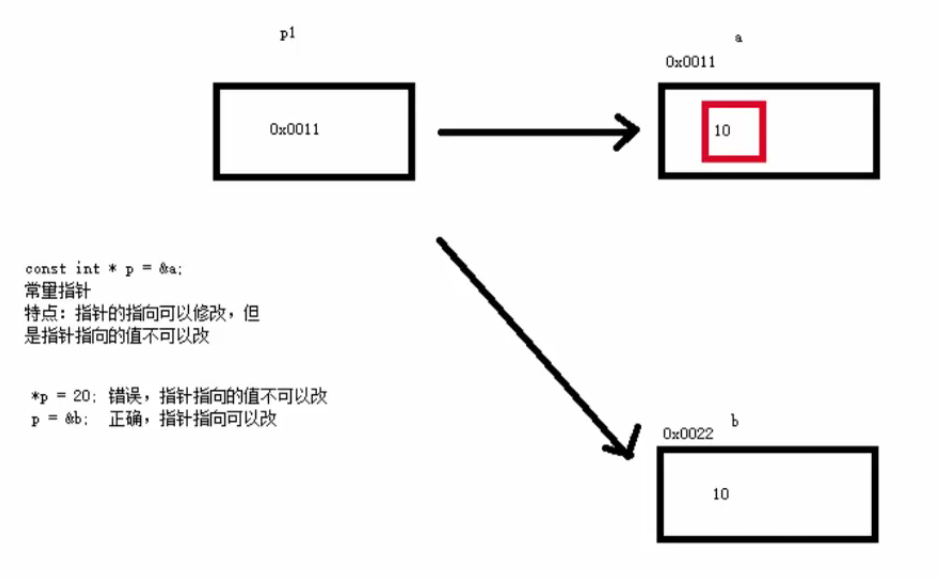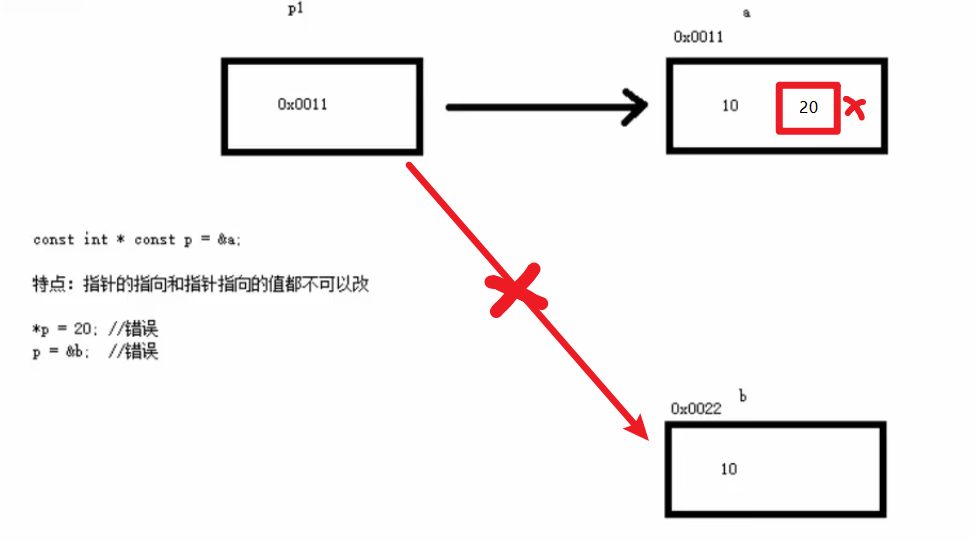WHCSRL 技术网

# 【C/C++】 常量指针，指针常量、指向常量的常指针详解

## 什么是指针

``````int a =10;
int*p;
p=&a; //把a的首地址赋值给P
```123```## 指针所占内存

32位系统中，处理器一次能处理的最大位数是2的32次方 ，也就是一次处理4个字节的内存数据，哪么指针的长度也就是4个字节

64位系统中，处理器一次能处理的最大位数是2的64次方 ，也就是一次处理8个字节的内存数据，哪么指针的长度也就是8个字节

32位系统指针4个字节 64位系统8个字节

## const 变量

const 是 constant 的缩写，本意是不变的，不易改变的意思

``````const int  a = 7;
int  b = a; // 正确
a = 8;       // 错误，不能改变
```123```

## 指针常量，常量指针和指向常量的指针

``````int * const p =&a;
```1```

``````const int *p=&a;
```1```

## 指针常量

``````int * const p =&a;
```1```

``````int a=10;
int * const p =&a;  //定义指针常量,指向int a的地址

*p = 20; //正确，指向的内存地址中的数据可以修改

p=&b;  //错误，指向的内存地址不可以修改
```123456```## 常量指针

``````const int *p=&a;
```1```

``````int a=10;
int b=10;
const int *p=&a;  //定义常量指针,指向int a的地址

*p = 20; //错误，指向的内存地址中的数据不可以修改

p=&b;  //正确，指向的内存地址可以修改
```1234567```## 指向常量的常指针

``````const int const *p=&a;
```1```

``````int a=10;
int b=10;

const int const *p=&a;  //定义指针常量,指向int a的地址

*p = 20; //错误，指向的内存地址中的数据不可以修改
p=&b;  //错误，指向的内存地址不可以修改
```1234567```## 空指针、野指针

``````//-------空指针-------//
int *p4 = NULL;
//cout << *p4 << endl; //运行Error，使用指针时必须先判断是否空指针
```123```

``````//-------野指针（悬浮、迷途指针）-------//

int *p5 =0x1100;  //任意赋值的野指针
//cout << *p5 << endl; //运行Error

int *p6 = new int(5);
delete p6;  //删除p6的指向
p6 = NULL; //一定要有这一步，否则p6会随机的指向一个地址

```12345678910```